## What is a Process in Python?

In computing, a process is an instance of a computer program that is being executed. Any process has 3 basic components:

• An executable program.
• The associated data needed by the program (variables, workspace, buffers, etc.)
• The execution context of the program (State of the process)

## An Intro to Python Threading

A thread is an entity within a process that can be scheduled for execution. Also, it is the smallest unit of processing that can be performed in an OS (Operating System). In simple words, a thread is a sequence of such instructions within a program that can be executed independently of other code. For simplicity, you can assume that a thread is simply a subset of a process! A thread contains all this information in a Thread Control Block (TCB):

• Thread Identifier: Unique id (TID) is assigned to every new thread
• Stack pointer: Points to the thread’s stack in the process. The stack contains the local variables under the thread’s scope.
• Program counter: a register that stores the address of the instruction currently being executed by a thread.
• Parent process Pointer: A pointer to the Process control block (PCB) of the process that the thread lives on.

Consider the diagram below to understand the relationship between the process and its thread: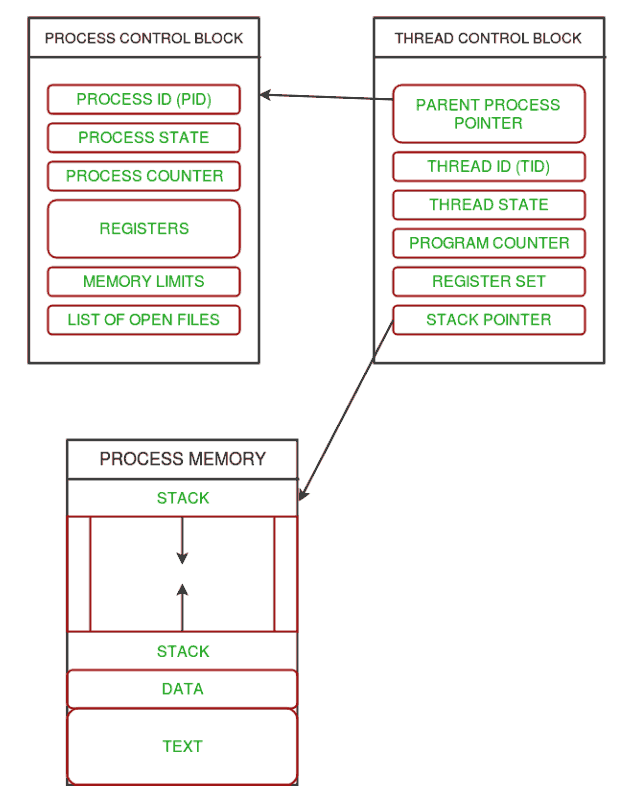Relationship between a Process and its Thread

Multiple threads can exist within one process where:

• Each thread contains its own register set and local variables (stored in the stack).
• All threads of a process share global variables (stored in heap) and the program code.

Consider the diagram below to understand how multiple threads exist in memory: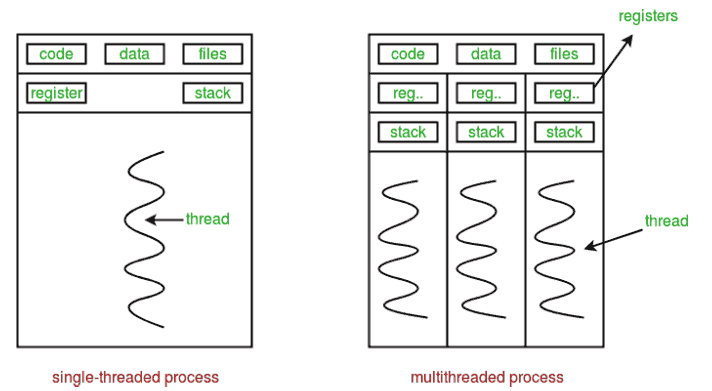Existence of multiple threads in memory

## An Intro to Threading in Python

Multithreading is defined as the ability of a processor to execute multiple threads concurrently. In a simple, single-core CPU, it is achieved using frequent switching between threads. This is termed context switching. In context switching, the state of a thread is saved and the state of another thread is loaded whenever any interrupt (due to I/O or manually set) takes place. Context switching takes place so frequently that all the threads appear to be running parallelly (this is termed multitasking).

Consider the diagram below in which a process contains two active threads: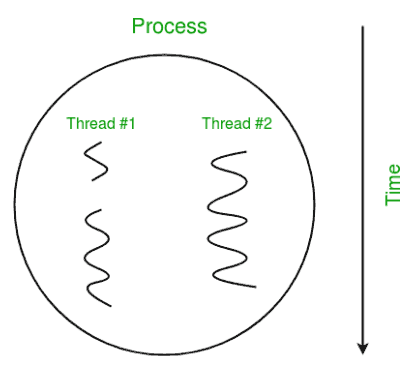In Python, the threading module provides a very simple and intuitive API for spawning multiple threads in a program. Let us try to understand multithreading code step-by-step.

Step 1: Import Module

`import threading`

To create a new thread, we create an object of the Thread class. It takes the ‘target’ and ‘args’ as the parameters. The target is the function to be executed by the thread whereas the args is the arguments to be passed to the target function.

`t1 = threading.Thread(target, args)t2 = threading.Thread(target, args)`

To start a thread, we use the start() method of the Thread class.

`t1.start()t2.start()`

Step 4: End the thread Execution

Once the threads start, the current program (you can think of it like a main thread) also keeps on executing. In order to stop the execution of the current program until a thread is complete, we use the join() method.

`t1.join()t2.join()`

As a result, the current program will first wait for the completion of t1 and then t2. Once, they are finished, the remaining statements of the current program are executed.

Example:

Let us consider a simple example using a threading module.

This code demonstrates how to use Python’s threading module to calculate the square and cube of a number concurrently. Two threads, `t1` and `t2`, are created to perform these calculations. They are started, and their results are printed in parallel before the program prints “Done!” when both threads have finished. Threading is used to achieve parallelism and improve program performance when dealing with computationally intensive tasks.

## Python3

 `import` `threading`     `def` `print_cube(num):` `    ``print``(``"Cube: {}"` `.``format``(num ``*` `num ``*` `num))`     `def` `print_square(num):` `    ``print``(``"Square: {}"` `.``format``(num ``*` `num))`     `if` `__name__ ``=``=``"__main__"``:` `    ``t1 ``=` `threading.Thread(target``=``print_square, args``=``(``10``,))` `    ``t2 ``=` `threading.Thread(target``=``print_cube, args``=``(``10``,))`   `    ``t1.start()` `    ``t2.start()`   `    ``t1.join()` `    ``t2.join()`   `    ``print``(``"Done!"``)`

Output:

`Square: 100Cube: 1000Done!`

Consider the diagram below for a better understanding of how the above program works: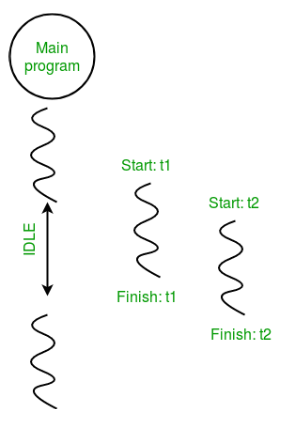Example:

In this example, we use os.getpid() function to get the ID of the current process. We use threading.main_thread() function to get the main thread object. In normal conditions, the main thread is the thread from which the Python interpreter was started. name attribute of the thread object is used to get the name of the thread. Then we use the threading.current_thread() function to get the current thread object.

Consider the Python program given below in which we print the thread name and corresponding process for each task.

This code demonstrates how to use Python’s threading module to run two tasks concurrently. The main program initiates two threads, `t1` and `t2`, each responsible for executing a specific task. The threads run in parallel, and the code provides information about the process ID and thread names. The `os` module is used to access the process ID, and the `threading'` module is used to manage threads and their execution.

## Python3

 `import` `threading` `import` `os`   `def` `task1():` `    ``print``(``"Task 1 assigned to thread: {}"``.``format``(threading.current_thread().name))` `    ``print``(``"ID of process running task 1: {}"``.``format``(os.getpid()))`   `def` `task2():` `    ``print``(``"Task 2 assigned to thread: {}"``.``format``(threading.current_thread().name))` `    ``print``(``"ID of process running task 2: {}"``.``format``(os.getpid()))`   `if` `__name__ ``=``=` `"__main__"``:`   `    ``print``(``"ID of process running main program: {}"``.``format``(os.getpid()))`   `    ``print``(``"Main thread name: {}"``.``format``(threading.current_thread().name))`   `    ``t1 ``=` `threading.Thread(target``=``task1, name``=``'t1'``)` `    ``t2 ``=` `threading.Thread(target``=``task2, name``=``'t2'``)`   `    ``t1.start()` `    ``t2.start()`   `    ``t1.join()` `    ``t2.join()`

Output:

`ID of process running main program: 1141Main thread name: MainThreadTask 1 assigned to thread: t1ID of process running task 1: 1141Task 2 assigned to thread: t2ID of process running task 2: 1141`

The diagram given below clears the above concept: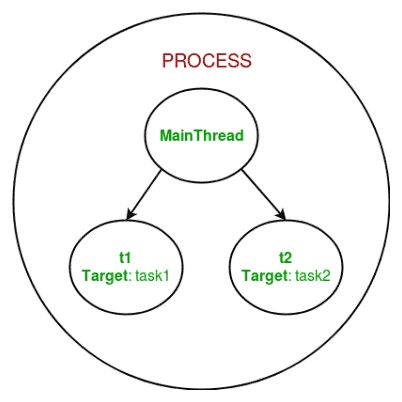So, this was a brief introduction to multithreading in Python. The next article in this series covers synchronization between multiple threads. Multithreading in Python | Set 2 (Synchronization)

A thread pool is a collection of threads that are created in advance and can be reused to execute multiple tasks. The concurrent.futures module in Python provides a ThreadPoolExecutor class that makes it easy to create and manage a thread pool.

In this example, we define a function worker that will run in a thread. We create a ThreadPoolExecutor with a maximum of 2 worker threads. We then submit two tasks to the pool using the submit method. The pool manages the execution of the tasks in its worker threads. We use the shutdown method to wait for all tasks to complete before the main thread continues.

This code uses a thread pool created with `concurrent.futures.ThreadPoolExecutor` to run two worker tasks concurrently. The main thread waits for the worker threads to finish using `pool.shutdown(wait=True)`. This allows for efficient parallel processing of tasks in a multi-threaded environment.

## Python3

 `import` `concurrent.futures`   `def` `worker():` `    ``print``(``"Worker thread running"``)`   `pool ``=` `concurrent.futures.ThreadPoolExecutor(max_workers``=``2``)`   `pool.submit(worker)` `pool.submit(worker)`   `pool.shutdown(wait``=``True``)`   `print``(``"Main thread continuing to run"``)`

Output

```Worker thread running